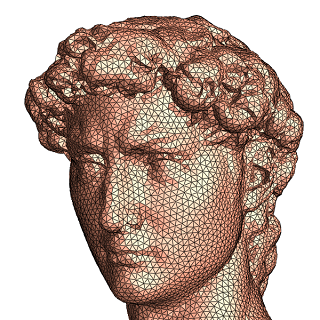# Harmoic Map Tutorial with C++ Source

### Smooth Case

Suppose $S$ is a surface embedded in the Euclidean space $R^3$. Furthermore, here we only consider the simplest topology: the surface $S$ is a topological disk. A topological disk is a surface homeomorphic to the planar unit disk, namely, an orientable, genus zero surface with a single boundary. The surface has an induced Euclidean metric $\mathbf{g}$. Suppose $f$ is a function from the surface to $R$. The harmonic energy of the function is defined as $$E(f) = \int_S |\nabla_{\mathbf{g}} f |^2 dA$$. The minimizer of the harmonic energy is called a harmonic function. A harmonic function satisfies the Laplace equation $\Delta_{\mathbf{g}} f = 0.$ with Dirichlet boundary condition $f|_{\partial S} = h,$ where $\partial S$ represents the boundary of the surface, $h$ is a given function. Suppose we choose an isothermal coordiante system $(u,v)$, then the Riemannian metric is represented as $\mathbf{g} = e^{2\lambda(u,v)} (du^2+dv^2),$ then the gradient operator is $\nabla_{\mathbf{g}} = e^{-\lambda(u,v)} (\frac{\partial}{\partial u},\frac{\partial}{\partial v})^T,$ the area element is $dA = e^{2\lambda(u,v)} du \wedge dv,$ and the Laplace-Beltrami operator is $\Delta_{\mathbf{g}} = e^{-2\lambda(u,v)} (\frac{\partial^2}{\partial u^2} + \frac{\partial^2}{\partial v^2}).$ Suppose $\phi:S \to D$ is a mapping, such that $\phi(u,v)=(x(u,v),y(u,v))$, both $x(u,v)$ and $y(u,v)$ are harmonic functions, then $\phi$ is called a harmonic map. Intuitively, we can imaginge the surface $S$ is made of rubber sheet, then we flattned the surface onto the planar unit disk, fixing the boundary. The mapping will naturally minimizes the membrane energy (stretching energy), and minizer is the harmonic map. Harmonic map is very useful for engineering applications, one fundamental reason is because harmonic map gives diffeomrophism under some appropriate conditions. Namely the mapping is bijective and smooth. This is formulated as Rado's theorem,

Rado's Theorem Suppose $\phi: S\to D$ is a harmonic map, $D$ is a planar convex domain. If the restriction of the map on the boundary is a homeomorphism, then the interior mapping is a diffeomorphism.

### Discrete Case

The surface is approximated by simplicial complex, namely triangle mesh $M$. A vertex is denoted as $v_i$; an oriented edge (halfedge) $[v_i,v_j]$, where $v_i$ is the source, $v_j$ is the target; an oriented face is $[v_i,v_j,v_k]$, where $v_i$, $v_j$ and $v_k$ are sorted counter-clock-wisely.A function $f: M\to R$ is defined on vertices. Suppose $p$ is a point inside a triangle $[v_i,v_j,v_k]$, then $p$ can be represented as the lineary combination of three vertices, $p = \lambda_i v_i + \lambda_j v_j + \lambda_k v_k,$ where $(\lambda_i,\lambda_j,\lambda_k)$ are called the barycentric coordinates of $p$, $\lambda_i + \lambda_j + \lambda_k = 1$. Barycentric coordinates can be computed as follows $\lambda_i = \frac{|[p,v_j,v_k]|}{|[v_i,v_j,v_k]|}$ where $|\cdot |$ represent the signed area of an oriented triangle. Then the function $f$ becomes a piece-wise linear function $f(p) = \lambda_i f(v_i) + \lambda_j f(v_j) + \lambda_k f(v_k).$ By direct computation, it is easy to show that the harmonic energy of the function $f$ can be written as $E(f) = \sum_{[v_i,v_j]\in M} w_{ij} (f(v_i) - f(v_j))^2.$ where $w_{ij}$ is called the cotangent weight on edge $[v_i,v_j]$. Suppose $[v_i,v_j]$ is adjacent to two faces $[v_i,v_j,v_k]$ and $[v_j,v_i,v_l]$, $\theta_k$ is the corner angle in $[v_i,v_j,v_k]$ with $v_k$ as the apex, similalary $\theta_l$ is the corner angle in $[v_j,v_i,v_l]$ at vertex $v_l$, then the edge weight $w_{ij} = \frac{1}{2}(\cot\theta_k + \cot \theta_l),$ if $[v_i,v_j]$ is an boundary edge, and only adjacent to one triangle $[v_i,v_j,v_k]$, then $w_{ij} = \frac{1}{2} \cot \theta_k.$ The discrete Laplace equation becomes, for all interior vertex $v_i \not\in \partial M$, $\sum_{[v_i,v_j]\in M} w_{ij} ( f(v_i) - f(v_j) ) = 0, \forall v_i\not\in \partial M,$ This is linar equation system.

### Algorithm

The computational algorithm is as follows:
1. Trace the mesh boundary, and get a sequence boundary vertices $\{v_0,v_1,\cdots, v_{n-1}\}$
2. Set the image of boundary vertex $v_i$ as $f(v_i) = (\cos\theta_i, \sin \theta_i), \theta_i = 2\pi \frac{\sum_{l=1}^i |v_{l}-v_{l-1}|}{\sum_{k=1}^n |v_{k}-v_{k-1}|},$
3. For all edges $[v_i,v_j]$ compute the cotangent edge weight $w_{ij}$ using the formula above.
4. For all invertior vertex $v_k$, go through all the surrounding vertices $v_j$, construct the discrete Laplace equation $\sum_{[v_k,v_j]\in M} w_{kj}(f(v_k)-f(v_j)) = 0,$
5. Solve the linear system.
The figure on top shows a discrete harmonic map result. Harmonic map is close to be conformal, so it preserves local shapes.

### Source Code

The code is written in C++ using VisualStudio on Windows platform.
If you encounter any problem during the compilation and running the code, please contact the author David Gu at $gu@cs.stonybrook.edu$.